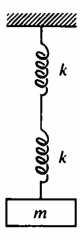# How to calc the period of this mass spring system?

LCSphysicist
Homework Statement:
How to calc the period of this mass spring system?
Relevant Equations:
All belowThis is a problem very easy to deal with if we consider the effective spring constant, however, i want to avoid this solution, and see how to justify the period of this motion just by analyse the forces or the energy, what seems a little hard to me.

First of all we would need to find the force that act on m, to do this we would need to find the stretched length of the spring touching the block, but to do this, we would need to know what is the displacement of the first spring too. ?

I don't know how to begin with it

Gold Member
First you should measure the position of the lowest end of the connected springs without the mass.
Then attach the mass, leave it at still and measure that position again.
mg / the difference of these positions = k of the connected springs. You will see it a half of k of one spring.

•LCSphysicist
PhysicsBoi1908
I was given this question by my teacher when I didn't know the effective spring constant.
My solution ended up deriving the effective spring constant by the end.
I think I assumed elongation in springs 1 and 2 to be ##x_1## and ##x_2## respectively, and then drew the free body diagrams for the joint of the springs and the block.
You might want to do this for springs with spring coefficients ##k_1 \text{ and } k_2## for more generality.

One more tip: When finding the period, you may want to assume that gravity doesn't exist to save some time.
The springs will have some initial elongation to account for the block's weight, so making this assumption would just result in the spring having no initial elongation. The period would remain the same.

•LCSphysicist